# Krzysztof Sikora

•### slope of a line segment

Activity

Krzysztof Sikora

•### locomotive

Activity

Krzysztof Sikora

•### equation of a circle: self-test 2

Activity

Krzysztof Sikora

•### approximations - significant figures: self test

Activity

Krzysztof Sikora

•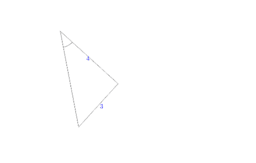### SOHCAHTOA: self test

Activity

Krzysztof Sikora

•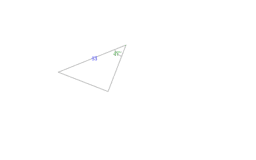### trigonometry - right triangles: self test

Activity

Krzysztof Sikora

•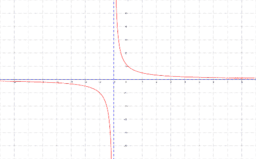### rational functions graphs: self-test

Activity

Krzysztof Sikora

•### polynomials: remainder theorem: self-test

Activity

Krzysztof Sikora

•### rational functions

Activity

Krzysztof Sikora

•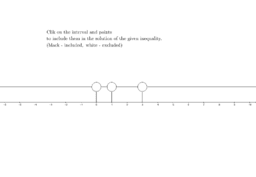### polynomial inequalities: self-test

Activity

Krzysztof Sikora

•### trigonometric equations: self-test

Activity

Krzysztof Sikora

•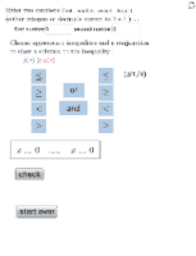Activity

Krzysztof Sikora

•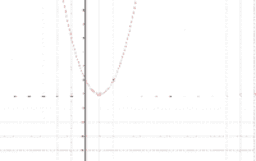### equation of a parabola - vertex form: self test

Activity

Krzysztof Sikora

•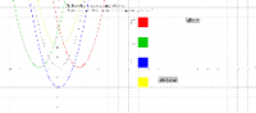### transformations of graphs: self-test 2

Activity

Krzysztof Sikora

•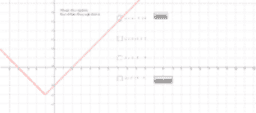### transformations of graphs: self-test 1

Activity

Krzysztof Sikora

•### approximations - decimal places: self-test

Activity

Krzysztof Sikora

•### slope of a straight line: self-test 2

Activity

Krzysztof Sikora

•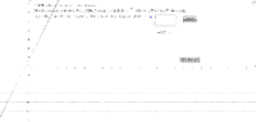### slope of a straight line: self-test 1

Activity

Krzysztof Sikora

•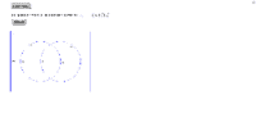### Venn diagrams: self-test

Activity

Krzysztof Sikora

•### is the number rational? self-test

Activity

Krzysztof Sikora

•### classification of numbers: self-test

Activity

Krzysztof Sikora

•Activity

Krzysztof Sikora

•### geometry

Book

Krzysztof Sikora

•### quadrilaterals - types and properties

Activity

Krzysztof Sikora